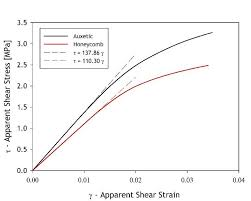## How to Calculate and Solve for Apparent Shear Stress | Slit Die | Polymer & TextileThe image above represents the apparent shear stress | slit die.

To compute for apparent shear stress | slit die, three essential parameters are needed and these parameters are height of die (h), pressure gradient (ΔP) and flow length (L).

The formula for calculating the apparent shear stress | slit die:

τapp = h . ΔP / 2L

Where:

τapp = Apparent Shear Stress | Slit Die
h = Height of Die
L = Flow Length

Let’s solve an example;
Find the apparent shear stress | slit die when the height of die is 7, pressure gradient is 12 and the flow length is 16.

This implies that;

h = Height of Die = 7
ΔP = Pressure Gradient = 12
L = Flow Length = 16

τapp = h . ΔP / 2L
τapp = 7 . (12) / 2(16)
τapp = 84 / 32
τapp = 2.625

Therefore, the apparent shear stress is 2.625.

Calculating the Height of Die when the Apparent Shear Stress, Pressure Gradient and the Flow Length is Given.

h = τapp x 2L / ΔP

Where:

h = Height of Die
τapp = Apparent Shear Stress | Slit Die
L = Flow Length

Let’s solve an example;
Find the height of die when the apparent shear stress | slit die is 32, the pressure gradient is 10 and the flow length is 8.

This implies that;

τapp = Apparent Shear Stress | Slit Die = 32
ΔP = Pressure Gradient = 10
L = Flow Length = 8

h = τapp x 2L / ΔP
h = 32 x 2 (8) / 10
h = 32 x 16 / 10
h = 512 / 10
h = 51.2

Therefore, the height of die is 51.2.